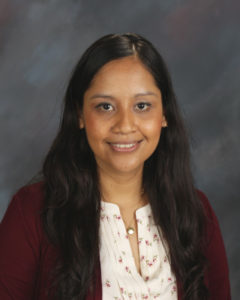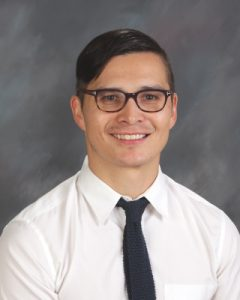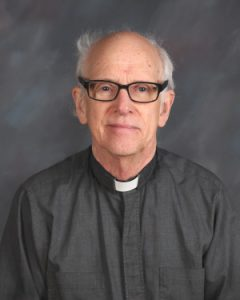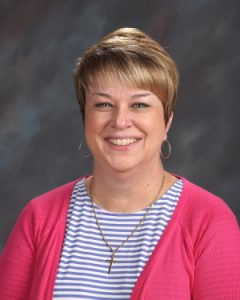# Mathematics

The usual course of study for a Loyola student is Algebra I, Geometry, Algebra II, Pre-Calculus, and Calculus. These courses are taught as college preparatory courses and the pace and levels of difficulty are set accordingly. For students with exceptional background and/or exceptional aptitude in math there are honors and AP options.

### Mathematics Graduation Requirements (6 semesters)

Students are required to complete six semesters of mathematics.  Prior to enrollment, students will take a placement exam to determine math aptitude, and course assignment.  Most students will be enrolled in an Algebra/Geometry hybrid course freshman year. Sophomore year students will complete Algebra II or Pre-Calculus, and junior year students take Pre-Calculus or Calculus.  Many seniors decide to enroll in a final year of math where they complete advanced placement courses in calculus, multivariable math, or computer programming/science.

### Faculty

#### Mathematics DepartmentSusan Torales
Department Chair, Mathematics x3048## Courses

#### Algebra 1 / Geometry

Teachers: Eli Ess
Open to: Array
Length: 4 Semesters

This one-year double-period course will teach students the basics of the number system and the use of algebraic expressions and equations. The course will cover functions, graphs, and applications. It will also develop the skills necessary to continue college-prep study. This course will provide a foundation, upon which students will build during their time here at Loyola.

Topics covered include: properties of real numbers; representation of verbal expressions as mathematical expressions; methods for solving equations, inequalities and application problems; proportions; factoring; simplifying rational and radical expressions; forms of linear equations and graphing techniques; and methods for solving systems of equations and quadratics.

The course also covers geometric terms and processes, logic, and problem solving. Various forms of proof are studied. Emphasis is placed upon reasoning and problem solving skills gained through study of the following major topics: angle relationships, congruent and similar triangles, geometric inequalities, parallel lines and their applications to quadrilaterals, areas of a polygon and circular regions, arcs and angle measures related to circles, and surface area and volume of solids. Trigonometry will be introduced as it applies to geometry. Algebraic skills (particularly work with ratios, simplification of and operations with radicals) are applied.

#### Accelerated Algebra 1 / Honors Geometry

Department: Mathematics
Teachers: Roger Yano, Emily Villa de Rey, Susan Torales, Judy Dell'Amico
Open to: Freshmen
Length: 4 Semesters

This one-year double-peiod course will teach students the basics of the number system and the use of algebraic expressions and equations. Students enrolled in this course are expected to already be proficient in applying the order of operations, performing operations with negative numbers and fractions, and solving equations and inequalities.

Topics covered include: proportions; factoring; simplifying rational and radical expressions; forms of linear equations and graphing techniques; and methods for solving systems of equations and quadratics.

In comparison to the college-preparatory level Geometry class, this honors course goes into greater depth and moves at a faster pace. This course covers geometric terms and processes, logic, and problem solving. Various forms of proof are studied. Emphasis is placed upon reasoning and problem solving skills gained through study of the following major topics: angle relationships, congruent and similar triangles, geometric inequalities, parallel lines and their applications to quadrilaterals, areas of a polygon and circular regions, arcs and angle measures related to circles, surface area and volume of solids, and coordinate geometry. Trigonometry will be introduced as it applies to geometry. Algebraic skills (particularly work with ratios, simplification of and operations with radicals) are applied.

#### Geometry

Teachers: Bren Wells
Open to: Freshmen

This course covers geometric terms and processes, logic, and problem solving. Various forms of proof are studied. Emphasis is placed upon reasoning and problem solving skills gained through study of the following major topics: angle relationships, congruent and similar triangles, geometric inequalities, parallel lines and their applications to quadrilaterals, areas of a polygon and circular regions, arcs and angle measures related to circles, surface area and volume of solids, and coordinate geometry. Trigonometry will be introduced as it applies to geometry. Algebraic skills (particularly work with ratios, simplification of and operations with radicals) are applied.

#### Geometry (Honors)

Department: Mathematics
Teachers: Emily Villa de Rey, Susan Torales, Zachary Sandoval, Judy Dell'Amico, Roger Yano
Open to: Freshmen
Length: 2 Semesters

This is a one-year course. Students at this level are expected to be self-motivated, independent learners. In comparison to the college-preparatory level Geometry class, this honors course goes into greater depth and moves at a faster pace. This course covers geometric terms and processes, logic, and problem solving. Various forms of proof are studied. Emphasis is placed upon reasoning and problem solving skills gained through study of the following major topics: angle relationships, congruent and similar triangles, geometric inequalities, parallel lines and their applications to quadrilaterals, areas of a polygon and circular regions, arcs and angle measures related to circles, surface area and volume of solids, and coordinate geometry. Trigonometry will be introduced as it applies to geometry. Algebraic skills (particularly work with ratios, simplification of and operations with radicals) are applied.

#### Algebra 2

Department: Mathematics
Teachers: Eli Ess, Chris Walter '93, Kelly Nicholson
Open to: Freshmen, Sophomores
Length: 2 Semesters

This one-year course will continue the student’s study of advanced algebraic concepts including a thorough discussion of the “elementary functions”: exponential, logarithmic and polynomial. Graphical and analytical thinking are stressed throughout the year, as is the development of problem solving skills. A programmable graphing calculator is recommended.

#### Algebra 2 (Honors)

Department: Mathematics
Teachers: Paul Pascale '78, Judy Dell'Amico, Ryan Abramowitz
Open to: Freshmen, Sophomores
Length: 2 Semesters

This is a one-year course. It is expected that students enrolled in this course will have completed Honors Geometry (or its equivalent) with a grade of B or better and have been recommended by their teacher for advancement in the Honors Program. A programmable graphing calculator is required. This course will explore the use of algebraic expressions, equations, and their graphical representations. Many of the topics covered in Algebra I will be reviewed and studied in greater depth, while some other topics will be introduced for the first time, including complex numbers and conic sections.

#### Pre Calculus

Department: Mathematics
Teachers: Rev. Jerry Hudson, SJ, Paul Pascale '78
Open to: Sophomores, Juniors
Length: 2 Semesters

This year-long course is for students who have successfully completed a full year of Algebra 2. Topics include: Trigonometric functions as ratios of sides of right triangles and as related to circular and simple harmonic motion; applications; methods for graphing; trigonometric identities, equations and formulas; inverse trigonometric functions; Law of Sines and of Cosines; trigonometric form of complex numbers and DeMoivre’s Theorem, exponential and logarithmic functions, including the natural logarithm; determinants and Cramer’s Rule; Binomial Theorem; sequences, including arithmetic and geometric; combinations, permutations and probability. A programmable graphing calculator is required.

#### Pre Calculus (Honors)

Department: Mathematics
Teachers: Zachary Sandoval, Susan Torales
Open to: Sophomores, Juniors
Length: 2 Semesters

This course is a continuation of Honors Algebra II. It is expected that students enrolled in this course will have completed Honors Algebra II (or its equivalent) with a grade of B or better and have been recommended by their teacher for advancement in the Honors Program. A scientific calculator is required, preferably a programmable one.

The emphasis in the first semester is on Trigonometry. Numerical methods, graphs, identities, and the inverse trigonometric functions are covered. Optional topics: vectors in 2 and 3 dimensions; and complex numbers in trigonometric form.

During the second semester, matrix algebra and linear systems are studied in some depth, with emphasis on applications. Polar and parametric graphs are also studied. An additional focus point is arithmetic, geometric, and recursive sequences and series, both finite and infinite. Optional topics include: Gaussian elimination; Cramer’s Rule; non-linear systems; mathematical induction; the binomial theorem; and elementary counting techniques (permutations and combinations).

#### Calculus

Department: Mathematics
Teachers: Matthew Baham, Ryan Abramowitz
Open to: Juniors, Seniors
Length: 2 Semesters

This one-year course consists of the first two semesters of Calculus. This course does not prepare the students for the AP exams, but will provide a good background for continued work in mathematics in college. Topics include: Graphical and numerical approaches to concepts of limits, derivatives and definite and indefinite integrals; techniques and properties of differentiation and integration; applications (optimization, related rates, rectilinear motion); exponential and logarithmic functions.

#### Statistics

Teachers: Ryan Abramowitz
Open to: Seniors

This year-long college preparatory course provides students with an introduction to probability and statistics. Topics include measures of central tendency, standard deviation, normal distributions, linear regression, the fundamental counting principle, conditional probability, permutations, and combinations. Throughout the year, the course will emphasize real-world applications and analysis.

#### Honors Multivariable Calculus

Teachers: Eric Romin
Open to: Seniors

This course is a study of the fundamental theorems and applications of multivariable calculus. It will extend the calculus of one-dimension–studied in the AP Calculus BC course–to Euclidean spaces of arbitrary dimension. The course will begin with a careful consideration of vectors in Rn. Next, we will consider the theory of partial derivatives and vector fields. Multiple Riemann integrals will then be considered as well as some of their applications. Time permitting, the course will conclude with a look at the important theorems of vector calculus – namely, the theorems of Green, Gauss, and Stokes.

#### AP Calculus AB

Department: Mathematics
Teachers: Emily Villa de Rey
Open to: Juniors, Seniors
Length: 2 Semesters

This is a one-year course, and one semester of college credit may be earned upon passing the A.P. Exam. Topics include: limits, continuity, differential and integral calculus, and related applications to include logarithmic, exponential, trigonometric functions. This course is restricted to students who have at least a 3.5 overall GPA and a 3.9 GPA in honors-level mathematics. Approval by the department is required. Honors Algebra II and Honors Pre-Calculus are the usual prerequisites.

#### AP Calculus BC

Department: Mathematics
Teachers: Eric Romin
Open to: Juniors, Seniors
Length: 2 Semesters

Calculus BC is a full-year course in the calculus of functions of a single variable. It includes all the topics covered in AP Calculus AB plus additional topics such as polar and parametric curves, differential equations and infinite series. Two semesters of college credit may be available upon passing the A.P. Exam. This course is restricted to students who have at least a 3.5 overall GPA and a 3.9 GPA in honors-level mathematics. Approval by the department is required. Honors Algebra II and Honors Pre-Calculus are the usual prerequisites.

#### AP Statistics

Department: Mathematics
Teachers: Brian Held '93
Open to: Seniors
Length: 2 Semesters

This one-year course is equivalent to a one-semester introductory non-calculus based college course in statistics. Students will be exposed to four broad conceptual themes: exploring data, planning a study, anticipating patterns, and statistical inferences. This course is restricted to students who have at least a 3.5 overall GPA and a 3.9 GPA in honors-level mathematics. Approval by the department is required. Honors Algebra II and Honors Pre-Calculus are the usual prerequisites for approval.

#### Computer Programming

Department: Mathematics
Teachers: Eric Romin
Open to: Juniors, Seniors
Length: 2 Semesters

This is a full-year for-credit introductory computer programming course that focuses on programming methodology and analysis using the Python programming language. Students will gain hands-on experience with all aspects of the development process through meaningful programming assignments. Topics include loops, decision-making structures, file processing, classes, object-oriented design, simple graphical user interfaces, data structures, and recursion. A strong mathematics background is desirable. Please note, this does not count as a mathematics course in terms of graduation requirements or college admissions.

#### AP Computer Science Principles

Department: Mathematics
Teachers: Eric Romin
Open to: Seniors
Length: 2 Semesters

AP Computer Science Principles introduces students to the foundational concepts of computer science and challenges them to explore how computing and technology can impact the world. Topics covered include programming, abstractions, algorithms, large data sets, the Internet, cybersecurity concerns, and computing impacts. With a unique focus on creative problem solving and real-world applications, AP Computer Science Principles prepares students for college and career.

#### AP Computer Science A

Department: Mathematics
Open to: Seniors
Length: 2 Semesters

This year course is designed to provide the student with a fundamental understanding of object- oriented programming using the Java language. AP Computer Science “A” covers program design, looping and decision making constructs, classes, inheritance hierarchies, polymorphism, and an introduction to recursion and data structures. This course is open to seniors with a strong mathematical background. Prior honor and/or AP math courses are desirable.

#### Mathematics Directed Study

A very few qualified students may sign a contract with the department agreeing to the amount of work they will do with a specified teacher. The students must draw up a program, have it approved by the directing teacher, and submit this to the department.﻿

#### Mathematics Teacher Aides

Teachers: Emily Villa de Rey

This program is for outstanding seniors who want to encounter the learning situation from a different viewpoint. The teacher aide is responsible for assisting the teacher in the daily classroom activities by leading group discussions and helping small groups in their work, and by assisting students who require tutorial help. The generous senior is given a chance to practice concern for his fellow man. Credit is given for this course. Students wishing to apply should consult with the teacher they wish to assist and then inform the Department Chairperson of their choice. This should be done weeks before registration. Prerequisite: Students must have a 3.0 GPA overall, a 3.0 GPA in Math, and a willingness to accept the responsibility of working in the classroom every day. Permission of the instructor and department chairperson is required.